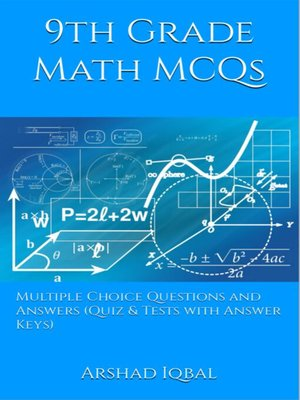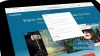### Multiple Choice Questions and Answers (Quiz & Tests with Answer Keys)

ebook

With an OverDrive account, you can save your favorite libraries for at-a-glance information about availability. Find out more about OverDrive accounts.

### Subjects

"9th Grade Math MCQs: Multiple Choice Questions and Answers (Quiz & Tests with Answer Keys)" provides practice tests for competitive exams. "Grade 9 Math MCQ" helps with theoretical, conceptual, and analytical study for self-assessment, career tests. This book can help to learn and practice 9th grade math quizzes as a quick study guide for placement test preparation.
"Grade 9 Math Multiple Choice Questions and Answers (MCQs)" pdf is a revision guide with a collection of trivia questions to fun quiz questions and answers pdf on topics: Algebraic expressions and algebraic formulas, algebraic manipulation, arithmetic and geometric sequences, basic math problems, basic statistics, business mathematics, congruent triangles and geometry, consumer math, factorization, introduction to logarithms, linear equations and inequalities, linear graphs and applications, logarithms and exponents, mathematical theorems, matrices and determinants, percentage, ratio and proportion, real and complex numbers, sets and functions to enhance teaching and learning.
Grade 9 Math Quiz Questions and Answers pdf also covers the syllabus of many competitive papers for admission exams of different schools from math textbooks on chapters:

Algebraic Expressions and Algebraic Formulas Multiple Choice Questions: 49 MCQs. Algebraic Manipulation Multiple Choice Questions: 13 MCQs. Arithmetic and Geometric Sequences Multiple Choice Questions: 36 MCQs. Basic Math Problems Multiple Choice Questions: 20 MCQs. Basic Statistics Multiple Choice Questions: 35 MCQs. Business Mathematics Multiple Choice Questions: 37 MCQs. Congruent Triangles and Geometry Multiple Choice Questions: 17 MCQs. Consumer Math Multiple Choice Questions: 20 MCQs. Factorization Multiple Choice Questions: 13 MCQs. Introduction to Logarithms Multiple Choice Questions: 30 MCQs. Linear Equations and Inequalities Multiple Choice Questions: 27 MCQs. Linear Graphs and Applications Multiple Choice Questions: 16 MCQs. Logarithms and Exponent.s Multiple Choice Questions: 12 MCQs. Mathematical Theorems Multiple Choice Questions: 24 MCQs. Matrices and Determinants Multiple Choice Questions: 48 MCQs. Percentage, Ratio and Proportion Multiple Choice Questions: 51 MCQs. Real and Complex Numbers Multiple Choice Questions: 61 MCQs. Sets and Functions Multiple Choice Questions: 30 MCQs.

The chapter "Algebraic Expressions and Algebraic Formulas MCQs" covers topics of algebraic expressions, algebra formulas, surds, rationalization of surds, and applications.
The chapter "Algebraic Manipulation MCQs" covers topics of square root of algebraic expression, basic mathematics, LCM, and HCF.
The chapter "Arithmetic and Geometric Sequences MCQs" covers topics of arithmetic sequence, arithmetic mean, sequence, geometric sequence, and geometric mean.
The chapter "Basic Math Problems MCQs" covers topics of math theorems, collinear points, distance formula, mid-point formula, Pythagoras theorem, and solving linear inequalities.
The chapter "Basic Statistics MCQs" covers topics of central tendency measurements, central tendency: mean, median and mode, measures of central tendency, cumulative frequency, frequency distribution, and measures of dispersion.
The chapter "Business Mathematics MCQs" covers topics of business partnership, discount formula, profit, and loss.
The chapter "Congruent Triangles and Geometry MCQs" covers topics of congruent triangles, construction of triangles, and mathematical definitions.
The chapter "Consumer Math MCQs" covers topics of personal income, and taxes.
The chapter "Factorization MCQs" covers topics of factorization, remainder theorem, and factor theorem.
The chapter...

### Publication Details

Publisher:
Imprint:
Smashwords Edition
Publication Date:
2017

### Format